## Arnold's Cat Map

The best known example of an Anosov Diffeomorphism. It is given by the Transformation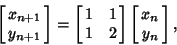(1)

where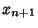and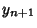are computed mod 1. The Arnold cat mapping is non-Hamiltonian, nonanalytic, and mixing. However, it is Area-Preserving since the Determinant is 1. The Lyapunov Characteristic Exponents are given by(2)

so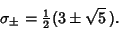(3)

The Eigenvectors are found by plugginginto the Matrix Equation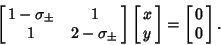(4)

For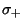, the solution is(5)

where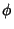is the Golden Ratio, so the unstable (normalized) Eigenvector is(6)

Similarly, for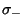, the solution is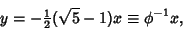(7)

so the stable (normalized) Eigenvector is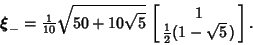(8)

See also Anosov Map

© 1996-9 Eric W. Weisstein
1999-05-25